# How to implement association rule algorithm? Python code and powerbi visualization are explained to you in detail (Part 2 - actual combat)

2022-02-01 19:46:14 28 dataIn the last one , I explained the principle and implementation steps of association rules , If you understand , It's easy to understand . But easier said than done , There are still problems in how to process the original data through tools to obtain effective and reliable results . The real job is to let you solve the problem, not just say the solution idea . This article is based on the theory , Show how to use... In combination with actual data Python Implement association rules and how to PowerBI Import Python The script generates the data table and displays it dynamically in a visual way .

In the use of Python When solving a problem , Actually, it's not from 0 To 1 Build it step by step , The process is cumbersome , Sometimes, in order to achieve a small effect, you may have to take a big turn , So it's like “ Diao Shen Xia ” equally , We often use other built ladders . That's why Python Language is so popular , Because it has a perfect open source community and countless tool libraries to achieve a certain purpose . When we implement the calculation of association rules , Using a machine learning library mlxtend Medium apriori,fpgrowth,association_rules Algorithm .
apriori Is a popular algorithm , It is used to extract frequent itemsets in association rule learning .apriori The algorithm aims to operate on the database containing transactions , For example, the purchase of store customers . If the user specified support threshold is met , Then the itemset is considered to be “ Frequent ”. for example , If the support threshold is set to 0.5 (50%), Then the frequent itemset is defined as at least 50% A set of items that appear together in all transactions of .

# One 、 Data sets

``````# Import related libraries
import pandas as pd
import mlxtend  # Machine learning library
# Coding package
from mlxtend.preprocessing import TransactionEncoder
# Association rule calculation package
from mlxtend.frequent_patterns import apriori, fpmax, fpgrowth,association_rules
pd.set_option('max_colwidth',150)  # Yes pandas Display effect settings , The maximum length of the column display field is 150 Characters           # Import dataset
Copy code ``````
``````# View the size of the dataset
Order.shape
Copy code ``````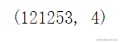``````# Before looking at the data 10 That's ok
Order.tail(5)
Copy code ``````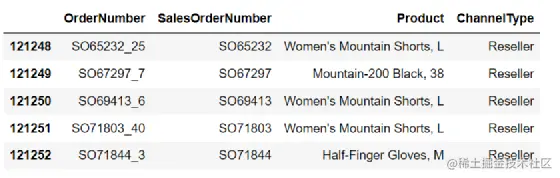There are 121253 Data , There are four fields in total ,SalesOrderNumber Refers to the order number ;ordernumber Refers to the sub order number , The next line change plus number indicates which sub order of the order , There may be one or more sub orders under each order number , And each sub order is not repeated , Corresponding to a product ;Product Refers to the trade name .

# Two 、mlxtend

Before we actually start, let's understand this mlxtend How to use the bag in . stay mlxtend The official website demonstrates how to realize the calculation of association rules , Let's take a look. There are three steps ：
First step , Import apriori Algorithm package ;
The second step , Process the original order data into the format supported by the algorithm ;
The third step , Calculate support 、 Degree of confidence 、 Improve the degree and other indicators and screen strong rules .

First step ： Import apriori Algorithm package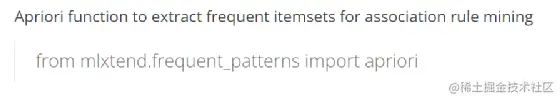The second step , Process the original order data into the format supported by the algorithm ：

The data actually obtained is often the same as the example in my last article , The goods are listed after the order number . therefore mlxtend The package accepts such a data format . But it can't be used directly for calculation , First, the real data is text , Two is apriori The algorithm package needs a method to convert the original data into commodity unique hot code Pandas Dataframe Format . The following is the input data ：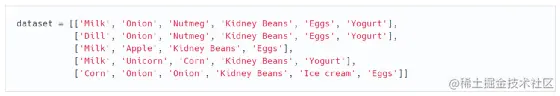It USES TransactionEncoder Package to convert the input data into the form we need . The following is the code and result of the conversion ：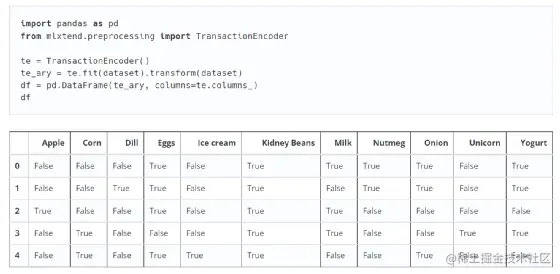Next , It feeds the processed data format to apriori Algorithm package , Calculate frequent itemsets （itemsets） And support . According to their preset minimum support is 0.6 To exclude infrequent itemsets .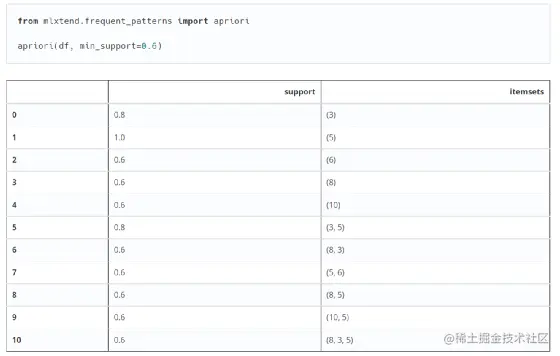However, there is a problem , The result set returned above is a number , It's actually df Each item in the table （item） The index of , This is more convenient for later processing , But if you just want to use this result . To increase readability , have access to use_colnames=True To display the original product name .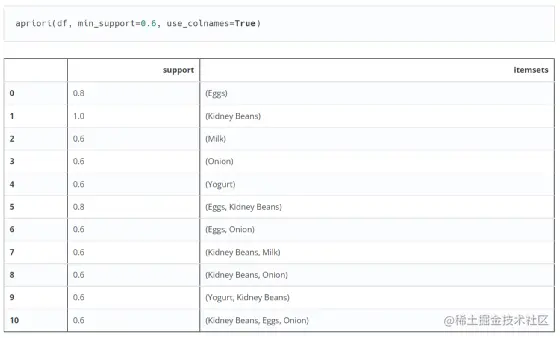The third step , Calculate support 、 Degree of confidence 、 Promotion

It's not here apriori The third step is demonstrated in the algorithm package , Why is that ？ because apriori The algorithm package is only the first step in association rules -- Find frequent itemsets , Of course, you won't find strong association rules ！ This step is in another package association_rules What's achieved in . This is also a problem that many beginners are prone to , I don't know the usage of each package , I don't know how to call the package .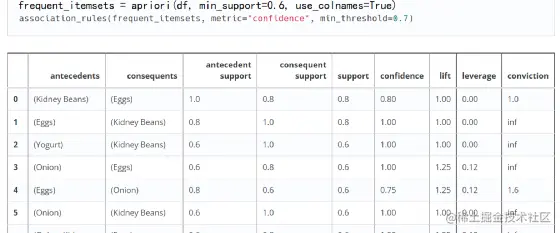In this way, we calculate the support of all frequent itemsets that meet the minimum support 、 Degree of confidence 、 Promotion , There are also leverage and conviction, These two functions are the same as the promotion degree lift It's the same thing , As I said before, it's best to use KLUC Measure and IR Unbalance ratio , But clearly mlxtend Most developers prefer to use leverage and conviction, I don't care about it here . This case only demonstrates the use of support lift.

# 3、 ... and 、Python Implement association rule code

## First step , Generate format data

The above is the usage of association rule package given on the official website . Next, I use my own data set to actually operate , And demonstrate how to PowerBI Import Python The script generates the data table and displays it dynamically in a visual way . The sample data has been given above . You can see that the dataset has multiple items per order , But line by line , Not an order followed by a line, so the form of goods . So here we need to find a way to convert from row to column . Use here Pandas Grouping function in , The effects to be achieved are as follows ：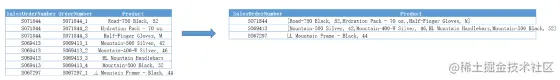``````# Use DataFrame Of groupby function
group_df = Order.groupby(['SalesOrderNumber'])
Copy code ``````

If you can write SQL We should know groupby Grouping must be used in conjunction with aggregation functions , Because it's a mapreduce The process of . If you group by order number without using aggregate function , stay SQL Server China will report an error , stay Mysql Only the first row of data will be returned . And here we use it directly groupby Grouping without aggregate function , Won't there be any problem ？ Let's take a look at the results group_df:

``````group_df.head(2) # Look at the dataset
Copy code ``````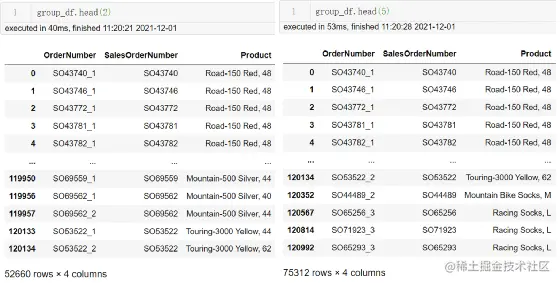The picture above shows groupby After the results of the （ I thought it was a data set ）, Before going to pick it up separately 2 And before 5 Let's see , The results show that the returned data and the size of the data set are different （ Note that the first thing we looked at the data showed was 121253 Data ）. Actually this groupby The result is a generator , It returns a dynamic grouping process , If you want to use the results, you can feed different parameters to get the actual results . So use this function to realize group_oncat, Generate product list .

``````df_productlist = pd.DataFrame({"productlist":group_df['Product'].apply(list)}).reset_index()
Copy code ``````

The above code means to generate a new table , This table is in accordance with SalesOrderNumber grouping , Then put the... Of each order group Product Aggregate according to the list ; This table has only two columns , One column is the grouping basis SalesOrderNumber, The other column is named productlist; Finally, remove the index of the original data object , Reset index , Finally get df_productlist surface , In this way, we form the input data format accepted by the algorithm . Here is the pre view 5 The result of that ：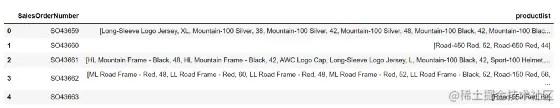``````# Because only frequent itemsets are needed , So remove the order number here , take productlist To numpy The array format .
df_array = df_productlist["productlist"].tolist()
Copy code ``````
``````df_array[0:3]  # Take the first three to see
Copy code ``````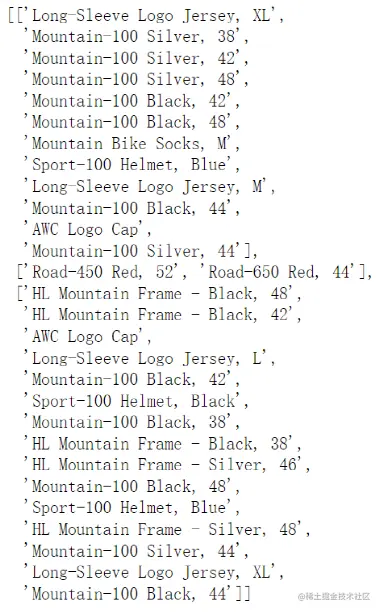You can see that the input data is formed , We'll use it again TransactionEncoder Process the data , Generate each item （ term ） The only hot code DataFrame Format ：

``````trans= TransactionEncoder()  # Call the encoding package
trans_array = trans.fit_transform(df_array)  # Feed the data set into the encoding package for conversion
df_item = pd.DataFrame(trans_array, columns=trans.columns_)  # Convert the converted data into DataFrame Format
Copy code ``````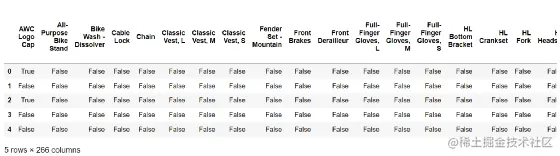## The second step , Generate frequent itemsets

After generating the final data format , Feed data to apriori Algorithm package generates frequent itemsets ：

``````# The minimum support given is 0.01, Show column names
frequent_itemset = apriori(df_item,min_support=0.01,use_colnames=True)
frequent_itemset.tail(5)
Copy code ``````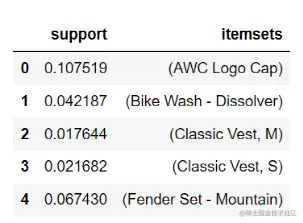The result of the algorithm is also DataFrame Frequent itemsets in format , It will make frequent 1 Itemsets 、 frequent 2 Itemsets 、 frequent 3 All results with minimum support greater than or equal to the itemset are returned . What is shown here is frequent 1 Itemsets .

In fact, in order to make it easier for us to view and filter , You can also count the length of frequent itemsets , This allows dynamic indexing .

``````frequent_itemset['length'] = frequent_itemset['itemsets'].apply(lambda x: len(x))
frequent_itemset[ (frequent_itemset['length'] == 2) &(frequent_itemset['support'] >= 0.01) ]
Copy code ``````

The meaning of this code is to find out that the support is greater than or equal to 0.01 Frequent 2 Itemsets , That is, the customer we often care about buys a commodity , What products will you buy .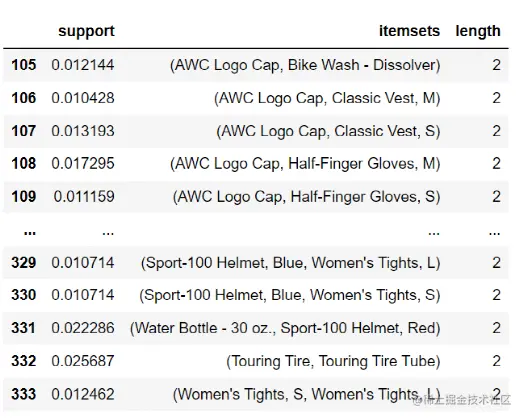After completing the first step of generating frequent itemsets , The following is mining association rules .

## The third step , Calculate support 、 Degree of confidence 、 Promotion

``````association = association_rules(frequent_itemset,metric="confidence",min_threshold=0.01)
Copy code ``````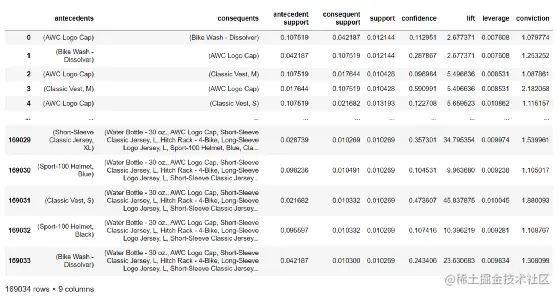The table above shows that a total of 169034 There are two alternative association rules , What do the names mean ？
antecedents： Indicates the product purchased first （ Combine ）,consequents Indicates the product purchased after （ Combine ）;
antecedent support： Indicates the product purchased first （ Combine ） Support of all orders ;
consequent support： Indicates the product purchased after （ Combine ） Support of all orders ;
support： It means buying two products at the same time （ Combine ） The situation accounts for the support of all orders ;
confidence： It means buying two products at the same time （ Combine ） The situation accounts for the support of all orders and antecedent support The ratio of the , That is, the confidence of the rule ;
lift： It means confidence And consequent support The ratio of the , That is, the degree of improvement , Verify the products purchased first （ Combine ） Buy again B The possibility and effectiveness of the product portfolio ;

Finally, we only focus on buying one and then another and buying two and then another , This is the most common actual scenario requirement . So generate two tables df_BuyAB and df_BuyABC. Here is the complete code , If you have data sets in the same format , You can run through this algorithm directly .

``````import pandas as pd
import mlxtend
from mlxtend.preprocessing import TransactionEncoder
from mlxtend.frequent_patterns import apriori, fpmax, fpgrowth,association_rules
group_df = Allorder.groupby(['SalesOrderNumber'])
df_productlist = pd.DataFrame({"productlist":group_df['Product'].apply(list)}).reset_index()
df_array = df_productlist["productlist"].tolist()
trans= TransactionEncoder()
trans_array = trans.fit_transform(df_array)
df_association = pd.DataFrame(trans_array, columns=trans.columns_)
frequent_itemset = apriori(df_association,min_support=0.01,use_colnames=True)
association = association_rules(frequent_itemset,metric="confidence",min_threshold=0.01)
BuyAB = association[(association['antecedents'].apply(lambda x :len(x)==1)) & (association['consequents'].apply(lambda x :len(x)==1))]
BuyABC = association[(association['antecedents'].apply(lambda x :len(x)==2)) & (association['consequents'].apply(lambda x :len(x)==1))]
Copy code ``````

The video at the beginning of the article shows how to use this Python The script realizes dynamic visualization , For the convenience of business personnel , Improve sales performance . If there is no video at the beginning of the article , You can go to my Zhihu to check .

Finally, you are welcome to pay attention to me , I'm Shilu , Search official account “ sixteen Data”, More technical dry goods continue to contribute .